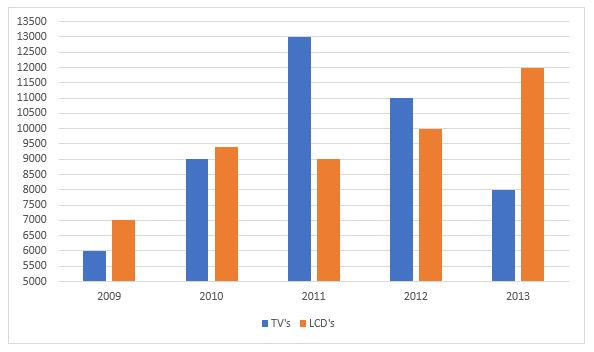SSC GD Quant Test 3

Instructions

Study the following bar diagram carefully and answer the following questions.

The number of the production of electronic items (TVs and LCDs) in a factory during the period from 2009 to 2013.Question 1

The ratio of production of LCDs in the year 2011 and 2013 is

Question 2

The difference between averages of production of TVs and LCDs from 2009 to 2012 is

Question 3

The ratio of production of TVs in the years 2009 and 2010 is

Question 4

The total number of products of electronic items is maximum in the year

Instructions

For the following questions answer them individually

Question 5

Find the value of $$\frac{4+3\sqrt{3}}{7+4\sqrt{3}}$$ is